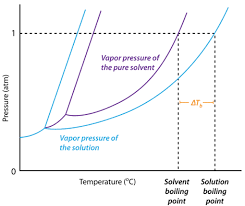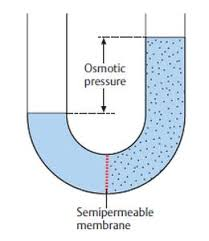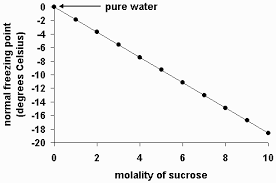## How to Calculate and Solve for Van’t Hoff Factor, Ebullioscopic Constant, Molality and Boiling Point Elevation | The Calculator EncyclopediaThe image above represents boiling point elevation.

To compute for the boiling point elevation, three parameters are needed and these parameters are Van’t Hoff’s Factor (i), ebullioscopic constant (Kb) and Molality.

The formula for calculating boiling point elevation:

δTb = iKb x Molality

Where;
δTb = boiling point elevation
i = Van’t Hoff’s Factor
Kb = ebullioscopic constant
Molality

Let’s solve an example;
Find the boiling point elevation when the Van’t Hoff’s Factor is 42, ebullioscopic constant is 60 and molality of 180.

This implies that;
i = Van’t Hoff’s Factor = 42
Kb = ebullioscopic constant = 60
Molality = 180

δTb = iKb x Molality
δTb = (42)(60) x 180
δTb = (2520) x 180
δTb = 453600

Therefore, the boiling point elevation is 453600 °C m-1.

Calculating the Molality using the Boiling Point Elevation, Van’t Hoff’s Factor and Ebullioscopic Constant.

Molality = δTb / iKb

Where;
Molality
δTb = boiling point elevation
i = Van’t Hoff’s Factor
Kb = ebullioscopic constant

Let’s solve an example;
Find the molality with a boiling point elevation of 120 and a van’t hoff’s factor of 32 with a ebullioscopic constant of 12.

This implies that;
δTb = boiling point elevation = 120
i = Van’t Hoff’s Factor = 32
Kb = ebullioscopic constant = 12

Molality = δTb / iKb
Molality = 120 / 384
Molality = 0.3125

Therefore, the molality is 0.3125.

## How to Calculate and Solve for Temperature, Number of Moles, Volume, Van’t Hoff Factor and Osmotic Pressure | The Calculator EncyclopediaThe image above represents the osmotic pressure.

To compute for the osmotic pressure, five parameters are needed and these parameters are Ideal Gas Constant (R)Temperature in Kelvin (T), Number of Moles (n), Volume (V) and Van’t Hoff’s Factor (i).

The formula for calculating osmotic pressure:

π = i nRTV

Where;
π = osmotic pressure
n = number of moles
R = ideal gas constant
T = temperature in Kelvin
i = Van’t Hoff’s Factor
V = Volume

Let’s solve an example;
Find the osmotic pressure when the ideal gas constant is 0.08206 with a temperature in kelvin of 120, number of moles is 32, a volume of 48 and a van’t hoff’s factor of 24.

This implies that;
n = number of moles = 32
R = ideal gas constant = 0.08206
T = temperature in Kelvin = 120
i = Van’t Hoff’s Factor = 24
V = Volume = 48

π = i nRTV
π = 24 32 x 0.08206 x 12048
π = (24) (315.110)(48)
π = (24)(6.5647)
π = 157.5

Therefore, the osmotic pressure is 157.5 atm.

Calculating the Van’t Hoff’s Factor using the Osmotic Pressure, Number of Moles, Temperature in Kelvin, Ideal Gas Constant and Volume.

i = / nRT

Where;
i = Van’t Hoff’s Factor
π = osmotic pressure
V = Volume
n = number of moles
R = ideal gas constant
T = temperature in Kelvin

Let’s solve an example;
Find the Van’t Hoff’s Factor when the osmotic pressure is 220, volume of 50, temperature in kelvin of 180 and number of moles of 60. (R = 0.08206)

This implies that;
π = osmotic pressure = 220
V = Volume = 50
n = number of moles = 60
R = ideal gas constant = 0.08206
T = temperature in Kelvin = 180

i = / nRT
i = 50 x 220 / 60 x 0.08206 x 180
i = 11000 / 866.808
i = 12.69

Therefore, the Van’t Hoff’s Factor is 12.69.

Calculating the Volume using the Osmotic Pressure, Number of Moles, Temperature in Kelvin, Ideal Gas Constant and Van’t Hoff’s Factor.

V = i (nRT) / π

Where;
V = Volume
i = Van’t Hoff’s Factor
π = osmotic pressure
n = number of moles
R = ideal gas constant
T = temperature in Kelvin

Let’s solve an example;
Find the volume when the osmotic pressure is 280, Van’t Hoff’s Factor of 40, temperature in kelvin of 90 and number of moles of 70. (R = 0.08206)

This implies that;
i = Van’t Hoff’s Factor = 40
π = osmotic pressure = 280
n = number of moles = 70
R = ideal gas constant = 0.08206
T = temperature in Kelvin = 90

V = i (nRT) / π
V = 40 (70 x 0.08206 x 90) / 280
V = 40 (516.978) / 280
V = 20679.12 / 280
V = 73.854

Therefore, the volume is 73.854.

## How to Calculate and Solve for Van’t Hoff’s Factor, Cryoscopic Constant, Molality and Freezing Point Depression | Nickzom CalculatorThe image above represents the freezing point depression.

To compute for the freezing point depression, three essential parameters are needed and these parameters are Van’t Hoff’s Factor (i), cryoscopic constant (Kf) and molality.

The formula for calculating freezing point depression:

δTf = iKf x Molality

Where;
δTf = Freezing point depression
i = Van’t Hoff’s Factor
Kf = cryoscopic constant
Molality

Let’s solve an example;
Find the freezing point depression when the van’t hoff’s factor is 12, cryoscopic constant is 21 with a molality of 16.

δTf = iKf x Molality
δTf = (12 x 21) x 16
δTf = 252 x 16
δTf = 4032

Therefore, the freezing point depression is 4032 °C m-1.